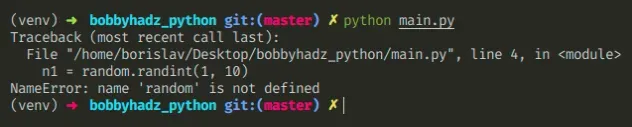# NameError: name 'random' is not defined in PythonLast updated: Apr 20, 2022Photo from Unsplash

## NameError: name 'random' is not defined in Python#

The Python "NameError: name 'random' is not defined" occurs when we use the `random` module without importing it first. To solve the error, import the `random` module before using it - `import random`.Here is an example of how the error occurs.

main.py
```Copied!```# ⛔️ NameError: name 'random' is not defined
n1 = random.randint(1, 10)

print(n1)

s1 = random.choice(['a', 'b', 'c'])
print(s1)
``````

To solve the error, we have to import the random module.

main.py
```Copied!```# ✅ import random module first
import random

n1 = random.randint(1, 10)

print(n1)

s1 = random.choice(['a', 'b', 'c'])
print(s1)
``````

Even though the `random` module is in the Python standard library, we still have to import it before using it.

Make sure you haven't used a capital letter `r` when importing `random` because module names are case-sensitive.

Also, make sure you haven't imported `random` in a nested scope, e.g. a function. Import the module at the top level to be able to use it throughout your code.

An alternative to importing the entire `random` module is to import only the functions that your code uses.

main.py
```Copied!```# 👇️ only import randint and choice functions
from random import randint, choice

n1 = randint(1, 10)

print(n1)

s1 = choice(['a', 'b', 'c'])
print(s1)
``````

The example shows how to import only the `randint` and `choice` functions from the `random` module.

Instead of accessing the members on the module, e.g. `random.randint()`, we now access them directly.

This should be your preferred approach because it makes your code easier to read.

For example, when we use an import such as `import random`, it is much harder to see which functions from the `random` module are being used in the file.

Conversely, when we import specific functions, it is much easier to see which functions from the `random` module are being used.

The `random` module is most commonly used to generate a random number from a range or select a random element from a sequence.

You can view all of the functions the `random` module provides by visiting the official docs.

## Conclusion#

The Python "NameError: name 'random' is not defined" occurs when we use the `random` module without importing it first. To solve the error, import the `random` module before using it - `import random`.

I wrote a book in which I share everything I know about how to become a better, more efficient programmer.You can use the search field on my Home Page to filter through all of my articles.